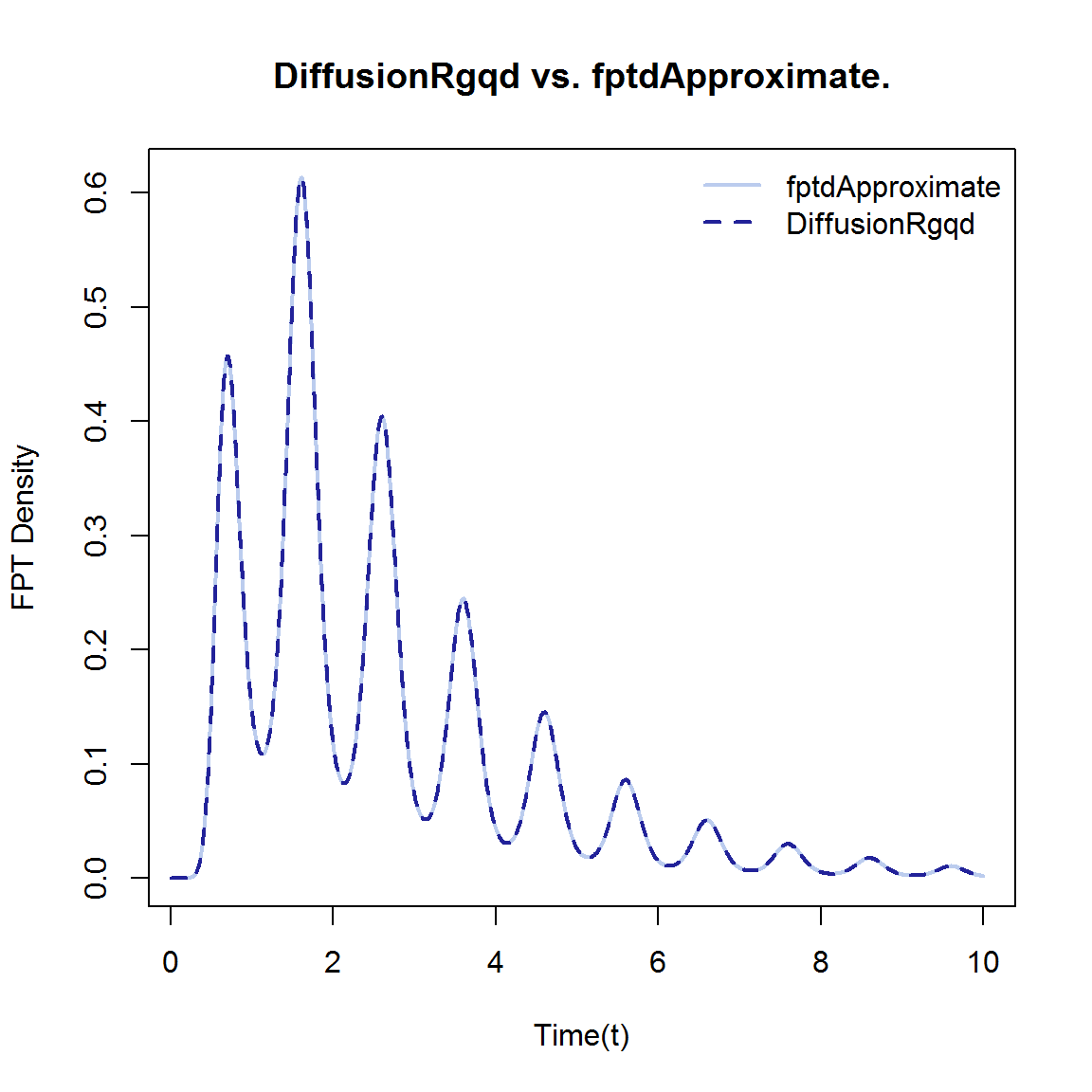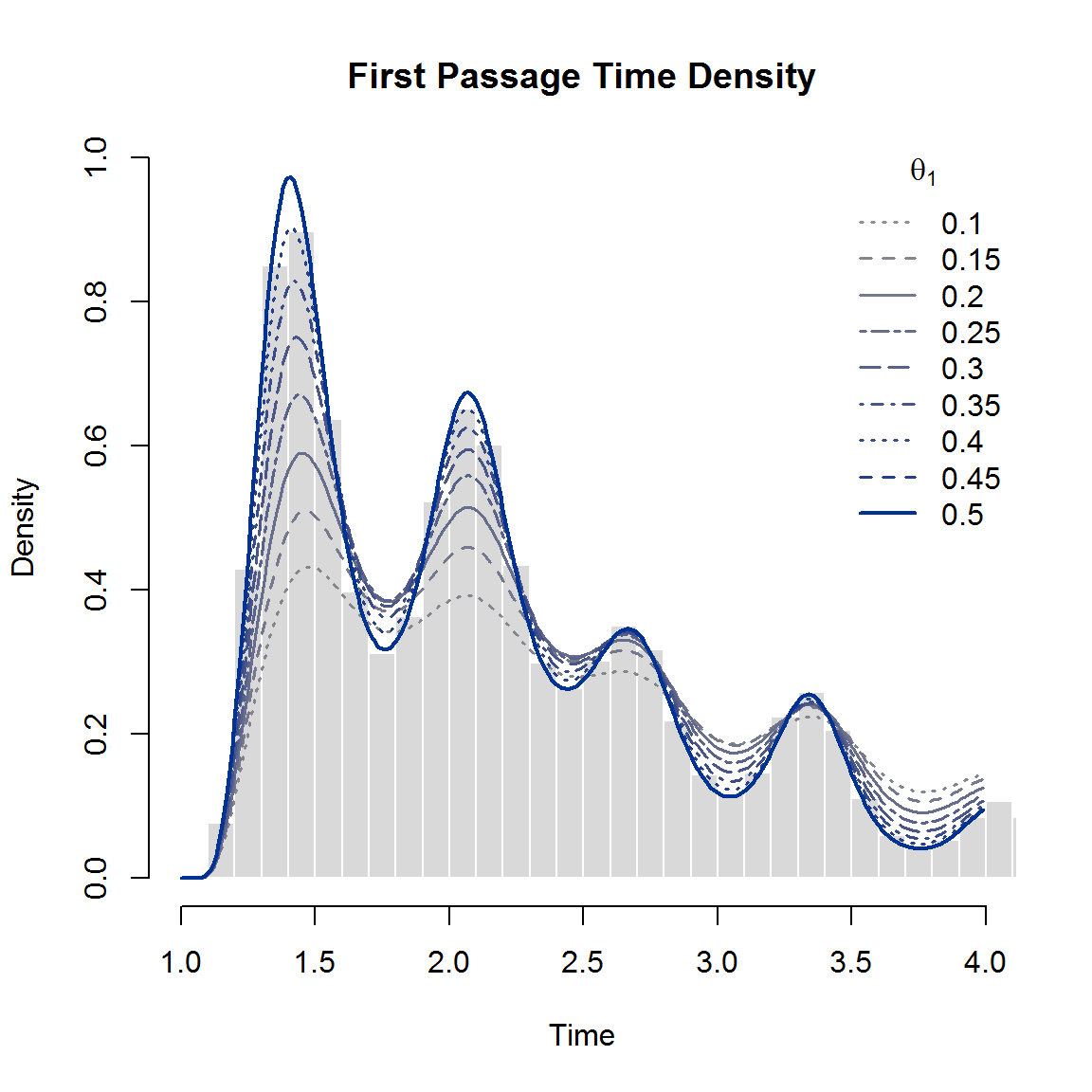# Scalar first passage time problems

Although most of the literature on diffusion processes are concerned with inferring dynamics from discretely observed trajectories, the application of diffusion models extend far beyond formulating a compact description of the dynamics of a given process. Often the objective of an analysis is to gain insight into the behaviour of events contingent on the trajectory of the process rather than the trajectory of the process itself. In a predictive context, one may be interested in the probability of a particular event occurring by a given time in the future. As such, a quantity that has been explored at length throughout the history of diffusion processes is the distribution of the time elapsed until the diffusion crosses a predefined threshold. The distribution of the first passage time $T_{X_s\rightarrow \lambda_t} = \inf\{t>s: X_t>\lambda_t\}$ of a scalar diffusion process $$X_t$$ crossing a fixed barrier $$\lambda_t$$ is given by the first kind Volterra equation $f(\lambda_t|X_s) = \int_s^t f(\lambda_t|\lambda_u)g(\lambda_u|X_s)du,$ where $$g(\lambda_t|X_s)$$ denotes the first passage time density evaluated at time $$t$$ and $$f(y|x)$$ is the transitional density of the diffusion process. Unfortunately, even when the transitional density is available exactly, the integral equation may not be analytically tractable. Fortunately, numerous techniques exist for evaluating the first passage time density by solving the integral equation numerically. Thus, by combining the cumulant truncation procedure for evaluating the transitional density with numerical procedures for evaluating the integral equation, we are able to evalaute first passage time problems for time-inhomogeneous generalized quadratic diffusions.

# Examples of time inhomogeneous first passage time problems

Another useful application of DiffusionRgqd package is the approximation of first passage time densities via the GQD.TIpassge() function. The GQD.TIpassage() function uses the same GQD interface as other functions in the package and operates in similar fashion to the GQD.density() function. However, since GQD.TIpassage() relies on calculating transitional density approximations for a large number of initial values in combination with the recursive updating algorithm of Buonocore, Nobile, and Ricciardi (1987), its internal workings have more in common with the GQD.mle() and GQD.mcmc() functions, where computationally optimized solutions with respect to the cumulant truncation procedure are constructed in C++ which is subsequently executed in R. As an introduction to the function we first compare the GQD.TIpassage() function to an existing R package for calculating first passage time densities for Gaussian diffusions and subsequently investigate the properties of the first passage time density of a non-linear diffusion transiting through a moving barrier.

An excellent package for the analysis of first passage time problems is the fptdApprox (Román-Román, Serrano-Pérez, and Torres-Ruiz 2014) package. Since fptdApprox can very effectively handle first passage time problems for diffusions with analytically tractable transitional densities we use it to compare some of the results from the DiffusionRgqd package. Consider for example a diffusion process with SDE:

$dX_t = 0.5(5-X_t)dt+dB_t,$ with $$X_0 =3$$. For purposes of calculating a first passage time consider then also a continuous barrier $\lambda_t = 5+0.25\sin(2\pi t).$ Under the fptdApprox package we may use the Approx.fpt.density() function in order to approximate the first passage time density. The interface requires that one define a diffusion process by configuring an object that consists of expressions giving the drift, diffusion and transitional density of the model at hand. The resulting object is then used by the Approx.fpt.density() function to approximate the first passage time density. More formally:

library("fptdApprox")
# Under fptdApprox':
# Define the diffusion process and give its transitional density:
OU <- diffproc(c("alpha*x + beta","sigma^2",
"dnorm((x-(y*exp(alpha*(t-s)) - beta*(1 - exp(alpha*(t-s)))/alpha))/
(sigma*sqrt((exp(2*alpha*(t-s)) - 1)/(2*alpha))),0,1)/
(sigma*sqrt((exp(2*alpha*(t-s)) - 1)/(2*alpha)))",
"pnorm(x, y*exp(alpha*(t-s)) - beta*(1 - exp(alpha*(t-s)))/alpha,
sigma*sqrt((exp(2*alpha*(t-s)) - 1)/(2*alpha)))"))
# Approximate the first passgage time density for OU, starting in X_0 = 3
# passing through 5+0.25*sin(2*pi*t) on the time interval [0,10]:
res1 <- Approx.fpt.density(OU, 0, 10, 3,"5+0.25*sin(2*pi*t)", list(alpha=-0.5,beta=0.5*5,sigma=1))
##
## Computing... Done.
##
## The value of the cumulative integral of the approximation is 0.992261204012805 < 1 - tol.
## If the value of the cumulative integral is not high and the final stopping instant is less than T, it may be appropriate:
##    - Check if the value of the final stopping instant increases using k argument to summary the fptl class object, or
##    - Approximate the density again with to.T = TRUE.

Using the __DiffusionRgqd__package we begin by defining the model as per usual according to the GQD framework. Then we call the GQD.TIpassage() function and provide it with the parameters of the first passage time problem. Note that GQD.TIpassage() circumvents the need to specify the transitional density as it will use the model coefficients to recognize and construct the appropriate numerical approximation of the required transitional densities. In present form, for computational purposes, the GQD.TIpassage() function evaluates the iterative updating equation of Buonocore, Nobile, and Ricciardi (1987) for the first passage time density density under constant barriers only. However, this is not a limiting constraint since for any barrier function that can be decomposed as $\lambda_t = \phi +h(t)$ where $$h(t)$$ is continuous, the first passage time problem $$\{Y_t = X_t - h(t) \rightarrow \phi| X_s-h(s)\}$$ is equivalent to that of $$\{X_t \rightarrow \lambda_t| X_s\}$$, provided that the transformed process is indeed a valid diffusion process. All that remains is to calculate the dynamics of $$Y_t$$ under Ito’s lemma.

library(DiffusionRgqd)
# Under DiffusionRgqd':
# Define the diffusion process
G0=function(t){0.5*5 - 0.5*pi*cos(2*pi*t) - 0.5*0.25*sin(2*pi*t)}
G1=function(t){-0.5}
Q0=function(t){1}
# Approximate the first passgage time density for OU, starting in X_0 = 3
# passing through 5+0.25*sin(2*pi*t) on the time interval [0,10]:
res2 <- GQD.TIpassage(Xs = 3, B = 5, s = 0, t = 10, delt = 1/200)
## Compiling C++ code. Please wait.


By plotting the subsequent approximations we infer from the resulting figure that the approximations are near identical.

# Let's compare the resulting densities:
plot(res1$y~res1$x, type = 'l', col = '#BBCCEE', xlab = 'Time(t)', ylab = 'FPT Density',
main = 'DiffusionRgqd vs. fptdApproximate.', lwd = 2)
lines(res2$density~res2$time, col = '#222299', lwd = 2, lty = 'dashed')
legend('topright', lty = c('solid', 'dashed'), col = c('#BBCCEE', '#222299'),
legend = c('fptdApproximate', 'DiffusionRgqd'), lwd = 2, bty = 'n')Note that since functions in the __DiffusionRgqd__always assumes that a numerical solution is required, it is possible for this example, where the transition density is available analytically, to produce a solution that is more efficiently calculable. However, the aim of the package is tackle problems with intractable dynamics under the GQD framework, where transition densities are rarely analytically available. Furthermore, as with the inference procedures, we again have a great deal of freedom for specifying a first passage time problem and subsequently calculating an accurate approximate solution. Consider for example a diffusion with SDE: $dX_t = \theta_1 X_t(10+0.2\sin(2\pi t)+0.3\sqrt{t}(1+\cos(3\pi t))-X_t)dt+\sqrt{0.1}X_tdB_t,$ with $$X_1 =8$$, $$\theta_1$$ a fixed parameter, and a constant barrier $$\lambda_t = 12$$. Note that for this SDE, no analytical solution for the transition density exists. Consequently, we can no longer make use of the fptdApprox package in order to generate the desired first passage time density, albeit for comparative purposes. As such we compare the resulting approximation to a simulated first passage time density for the quadratic diffusion transiting through $$\lambda_t = 12$$. Perhaps the simplest algorithm for achieving this is to simulate numerous trajectories of the diffusion and record the times at which these trajectories cross $$\lambda_t$$. In R this can be achieved for example by:

# Simulate the first passage time density:
theta <- 0.5
mu <- function(X,t){theta*(10+0.2*sin(2*pi*t)+0.3*sqrt(t)*(1+cos(3*pi*t)))*X-theta*X^2}
sigma <- function(X,t){sqrt(0.1)*X}
simulate.fpt=function(N,S,B,delt)
{
X=rep(S,N)
Ndim=N
time.vector=rep(0,N)
t=1
k1=0
while(Ndim>=1)
{
X=X+mu(X,t)*delt+sigma(X,t)*rnorm(Ndim,sd=sqrt(delt))
# Check if the barrier is crossed and keep trajectories that
# have survived:
I0=X<B
X=X[I0]
count=sum(!I0)
Ndim=length(X)
t=t+delt
if(count>0)
{
time.vector[k1+1:count]=t
k1=k1+count
}
}
return(time.vector)
}

fpt.sim.times <- simulate.fpt(500000, 8, 12, 1/2000)

where we have set $$\theta_1 =0.5$$. It is important to note that this scheme is subject to bias since the finite step size used in the simulation implies that the scheme fails to account for trajectories that may have already crossed $$\lambda_t$$ yet are recorded below $$\lambda_t$$ subsequent to each update (Giraudo and Sacerdote 1999). However this is a somewhat technical matter which falls outside of the scope of the present paper. As such we have chosen the parameters of the diffusion in such a way that the scheme suffices for visual comparison. Using GQD.TIpassage() we can again analyse the first passage time problem by defining the model in terms of GQD-coefficients:

GQD.remove()
##  "Removed :  G0 G1 Q0"
# Redefine the coefficients with a parameter theta:
G1 <- function(t){theta*(10+0.2*sin(2*pi*t)+0.3*prod(sqrt(t),1+cos(3*pi*t)))}
G2 <- function(t){-theta}
Q2 <- function(t){0.1}
# Now just give a value for the parameter in the standard fashion:
res3=GQD.TIpassage(8,12,1,4,1/100,theta=c(0.5))
## Compiling C++ code. Please wait.


Note that we have parametrised the coefficients using the reserved variable theta in similar fashion to BiGQD.mcmc(). This allows one to calculate the first passage time density for various parameter values without having to re-compile C++ code repeatedly. For example, we can evaluate the first passage time problem for the quadratic diffusion for $$\theta_1$$ running from $$0.1$$ to $$0.5$$:

# Load simulated first passage times: fpt.sim.times'
data("SDEsim6")
hist(fpt.sim.times, freq = F, col = 'gray85', border = 'white',
main = 'First Passage Time Density', ylab = 'Density', xlab = 'Time',
ylim = range(res3$density), xlim = range(res3$time), breaks = 100)

library("colorspace")
colpal = function(n){rev(sequential_hcl(n, power = 1, l = c(20, 60)))}
th.seq = seq(0.1, 0.5, 0.05)
for(i in 2:length(th.seq))
{
res3 = GQD.TIpassage(8, 12, 1, 4, 0.01, theta = c(th.seq[i]))
lines(res3$density ~ res3$time, type = 'l', col = colpal(10)[i],
lty = 11 - i, lwd =1.5)
}
## Compiling C++ code. Please wait.

Compiling C++ code. Please wait.

Compiling C++ code. Please wait.

Compiling C++ code. Please wait.

Compiling C++ code. Please wait.

Compiling C++ code. Please wait.

Compiling C++ code. Please wait.

Compiling C++ code. Please wait.

lines(res3$density ~ res3$time, type = 'l', col = colpal(10)[i], lwd = 2)
legend('topright', legend = th.seq, col = colpal(10), lty = 9:1,
lwd = c(rep(1.5, 8), 2), title = expression(theta), bty = 'n')The resulting figure illustrates the effect of varying $$\theta_1$$: As the value of the parameter decreases the time taken to reach and exceed the barrier increases and the effect of the time dependent terms become less prominent. This makes sense since $$\theta_1$$ in some sense dictates the speed’ at which the process drifts toward the equilibrium line $$10+0.2\sin(2\pi t)+0.3\sqrt{t}(1+\cos(3\pi t)$$. Since this line starts out above the starting point $$X_1 =8$$, $$\theta_1$$ will dictate how intensely the drift of the process pulls it towards the barrier. Finally, comparing the approximate first passage time density to that of the simulated first passage time, it can be seen that the approximation is indeed valid.

# Further reading

browseVignettes('DiffusionRgqd')